# GED Math : Decimal and Fraction Conversions

## Example Questions

← Previous 1

### Example Question #1 : Decimal And Fraction Conversions

Express as a fraction: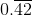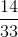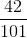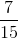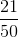Explanation:

Let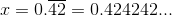Then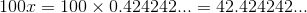Subtract: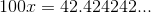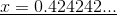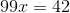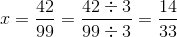### Example Question #2 : Decimal And Fraction Conversions

Express as a decimal: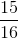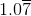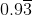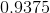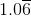Explanation:

Divide 15 by 16: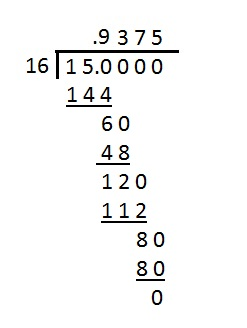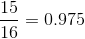### Example Question #3 : Decimal And Fraction Conversions

Express as a fraction: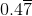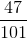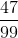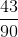Explanation:

Let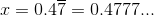Then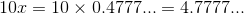and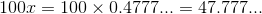We can subtract: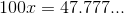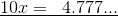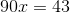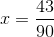### Example Question #4 : Decimal And Fraction Conversions

Which number has 5.25 as its reciprocal?

Do not use a calculator.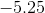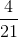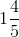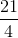Explanation:

The number that has 5.25 as its reciprocal is, in return, the reciprocal of 5.25.

Convert 5.25 to a fraction: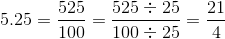Switch the numerator and the denominator to obtain the reciprocal - this number is.

### Example Question #5 : Decimal And Fraction Conversions

Which of the following numbers has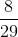as its reciprocal?

Do not use a calculator.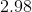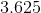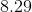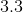Explanation:

The number that hasas its reciprocal is, in return, the reciprocal of. This number is the result of switching the numerator and denominator -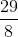. Since we are looking for the decimal equivalent, we divide 29 by 8: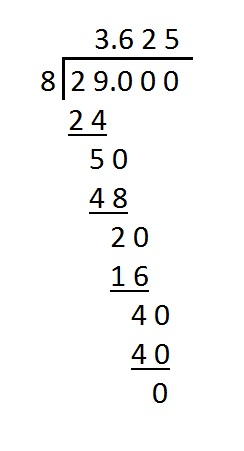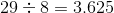, the correct choice.

### Example Question #6 : Decimal And Fraction Conversions

Express as a decimal: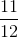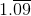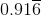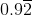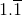Explanation:

Divide 11 by 12: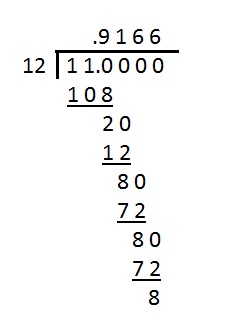The "6" repeats forever,  so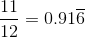.

### Example Question #7 : Decimal And Fraction Conversions

Express as a decimal: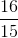Explanation:

Divide 16 by 15: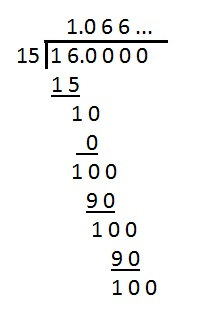The "6" repeats forever, so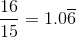.

### Example Question #8 : Decimal And Fraction Conversions

Express as a decimal: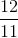Explanation:

Divide 12 by 11: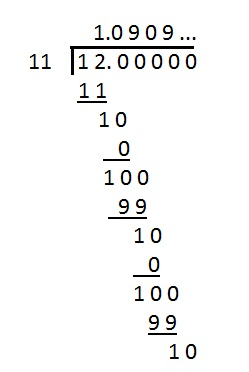The "09" repeasts forever, so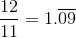.

### Example Question #9 : Decimal And Fraction Conversions

What is the decimal represented by the fraction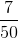?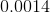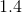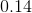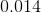Explanation:

Set up a proportion such that this fraction is some number over 100.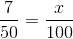Cross multiply.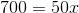Divide by 50 on both sides.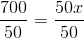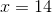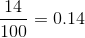The answer is:### Example Question #10 : Decimal And Fraction Conversions

Rewrite 0.24 as a fraction in simplest form.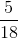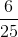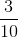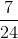Explanation:

The last nonzero digit of 0.24 - namely, the "4" - is in the hundredths place, so the fractional representation is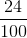Reduce this to simplest form by dividing both halves by their greatest common factor, which is 4: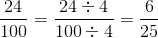← Previous 1